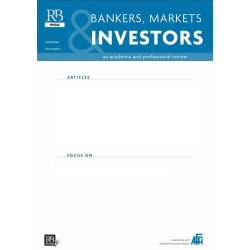Agrandir

# Estimation Risk versus Optimality Risk: [extrait BMI 132]

En Savoir Plus

47,39 €

BMI132-1118858

Version numérique PDF

Implementing portfolio optimization techniques is a challenging task because of the presence of estimation risk in expected return and covariance parameters. This paper provides an empirical analysis of the tradeoff between estimation risk, which is the risk of imperfectly estimating the parameters required for optimization, and optimality risk, which is the risk of selecting a weighting scheme that is a priori inferior to the maximum Sharpe ratio (MSR) portfolio but requires fewer parameter estimates, and as such is less impacted by the presence of estimation risk. We first show that if parameters were perfectly known, all weighting schemes wouldinvolve a substantial loss of efficiency with respect to the MSR portfolio. We also find that the risk parity portfolio involves the lowest efficiency loss amongst all analyzed weighting schemes. The introduction of estimation risk does not alter the domination of the MSR portfolio if the true underlying asset pricing model is known to the investor. In the presence of model risk, on the other hand, the MSR portfolio does no longer achieve the highest ex-ante Sharpe ratio, and is dominated by a number of alternative strategies, including the risk parity weighting scheme, as well as the inverse volatility and the inverse variance weighting schemes, which prove attractive when small samples are used.JEL Codes: G11.Keywords: Alternative Equity Indexation; Efficient Portfolio; Parameter Uncertainty.

Auteurs :Martellini Lionel
Extrait de la revue BMI 132

Aucun commentaire n'a été publié pour le moment.

## Donner votre avisEstimation Risk versus Optimality Risk: [extrait BMI 132]